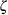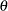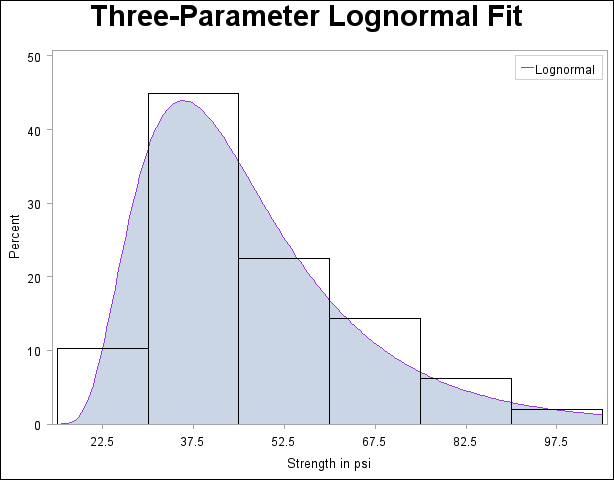The UNIVARIATE Procedure

## Example 4.24 Fitting a Three-Parameter Lognormal Curve

If you request a lognormal fit with the LOGNORMAL primary option, a two-parameter lognormal distribution is assumed. This means that the shape parameterand the scale parameterare unknown (unless specified) and that the thresholdis known (it is either specified with the THETA= option or assumed to be zero).

If it is necessary to estimatein addition toand, the distribution is referred to as a three-parameter lognormal distribution. This example shows how you can request a three-parameter lognormal distribution.

A manufacturing process produces a plastic laminate whose strength must exceed a minimum of 25 pounds per square inch (psi). Samples are tested, and a lognormal distribution is observed for the strengths. It is important to estimateto determine whether the process meets the strength requirement. The following statements save the strengths for 49 samples in the data set Plastic:

```data Plastic;
label Strength = 'Strength in psi';
input Strength @@;
datalines;
30.26 31.23 71.96 47.39 33.93 76.15 42.21
81.37 78.48 72.65 61.63 34.90 24.83 68.93
43.27 41.76 57.24 23.80 34.03 33.38 21.87
31.29 32.48 51.54 44.06 42.66 47.98 33.73
25.80 29.95 60.89 55.33 39.44 34.50 73.51
43.41 54.67 99.43 50.76 48.81 31.86 33.88
35.57 60.41 54.92 35.66 59.30 41.96 45.32
;
run;
```

The following statements use the LOGNORMAL primary option in the HISTOGRAM statement to display the fitted three-parameter lognormal curve shown in Output 4.24.1:

```title 'Three-Parameter Lognormal Fit';
proc univariate data=Plastic noprint;
histogram Strength / lognormal(fill theta = est noprint);
inset lognormal    / format=6.2 pos=ne;
run;
```

The NOPRINT option suppresses the tables of statistical output produced by default. Specifying THETA=EST requests a local maximum likelihood estimate (LMLE) for, as described by Cohen (1951). This estimate is then used to compute maximum likelihood estimates forand.

Note:You can also specify THETA=EST with the WEIBULL primary option to fit a three-parameter Weibull distribution.

A sample program for this example, uniex14.sas, is available in the SAS Sample Library for Base SAS software.

Output 4.24.1 Three-Parameter Lognormal FitPrevious Page | Next Page | Top of Page